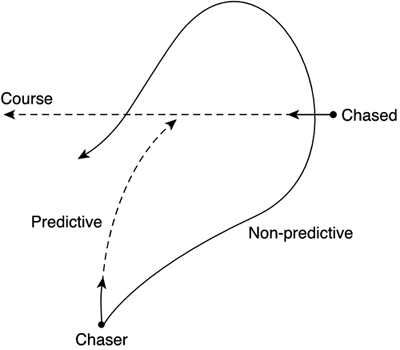# Chasing

Now that we know how to aim at a target, we will use that knowledge to implement a chase behavior. To be honest, chasing is easy once you know how to keep eye contact, so this section should be pretty straightforward.

In its simplest form, chasing involves moving forward while keeping eye contact with the target. Thus, we will keep aligned with the target and advance toward it. If by some unknown reason we lose sight of the target (because the target moved, for example), we will correct our orientation and keep moving.

#### Chase 2D: Constant Speed

The source code for a constant-speed, 2D chaser follows. Notice how we follow the guideline in the previous section, and just try to re-aim while moving forward. More sophisticated, variable-speed methods can be devised for specific purposes.

` void chase(point mypos, float myyaw, point hispos) { reaim(mypos,myyaw,hispos); mypos.x = mypos.x + cos(myyaw) * speed; mypos.z = mypos.z + sin(myyaw) * speed; } `

The success of this approach largely depends on the relationship between our speed, the target's speed, and our turning ability. If we can turn quickly, we can assume that we will be effectively facing the target much of the time, so we will perform a pretty optimal chase. But we will need our speed to be higher than the target's speed to ensure we make contact. A faster target will thus be unreachable. On the other hand, a much slower target might also escape our pursuit, especially if our maneuverability is restricted. To understand this, think of a fighter jet trying to chase a helicopter. Quite likely, the jet will have a hard time trying to stay aligned with the helicopter.

#### Predictive Chasing

One alternative to ensure a better chase is to use predictive techniques. Here we will not aim at the target directly, but try to anticipate his movements and guess his intentions. In much the same way as a good chess player can use intuition to discover what his opponent is trying to do, a chaser can use some clever interpolation to anticipate his opponent's moves.

This idea is really straightforward. Keep track of the position history of the opponent and use that information to create a "predicted position" some time in the future. Then, aim at that position. In Figure 7.4, you can see how a predictive chaser can outperform an opponent, even if their respective speeds and maneuverability are the same. The predictive chaser will make more informed decisions, which will ultimately make him succeed.

##### Figure 7.4. Predictive chasing, where the dotted line shows the predictive course.Predictive chasing is performed as a preprocess to the chase routine just explained. Instead of aiming and advancing, we will use a three-step approach, which involves:

1. Calculating a projected position

2. Aiming at that position

Calculating the projected position can be implemented with a number of interpolation techniques. We could, for example, take the last two position samples from the enemy and use a straight line as the interpolator. The code for this approach would look something like this:

` void chase(point mypos, float myyaw, point hispos,point prevpos) { point vec=hispos-prevpos;      // vec is the 1-frame position difference vec=vec*N;                     // we project N frames into the future point futurepos=hispos+vec;    // and build the future projection reaim(mypos,myyaw,futurepos); mypos.x = mypos.x + cos(myyaw) * speed; mypos.z = mypos.z + sin(myyaw) * speed; } `

Just these three simple lines allow our chaser to perform better. By varying the value of N (which depends on the relationships of speeds and our chaser's turning ability), we can find the perfect match.

An added value of the preceding code is that it will correctly treat the degenerate case of a target that holds still. It will predict that the target will remain unchanged.

We can implement variants of the preceding code using better interpolators. Instead of using the last two points in the trajectory, we could use N points for better results. By using N points, we can derive an N-1 interpolation polynomial. Obviously, as we increase the degree of the polynomial, the fitness to the trajectory will improve, and we will be able to make longer-lived predictions. But we will soon see that this improvement comes at a price. Computing higher-order polynomials has a computational cost that you might not be willing to pay.

One of the best ways to compute interpolation polynomials is to use the method of finite differences. These are a set of equations that define the Nth degree polynomial (thus, passing through N+1 values). For a quadratic polynomial, the equation has the form:

` P(x) = a0 + a1*(X-x0) + a2*(X-x0)*(X-x1) `

where:

` a0 = y1 a1 = (y1   y0) / (x1-x0) a2 = (((y2   y1) / (x2-x1)) - ((y1-y0) / (x1-x0))) / (x2-x0) `

You can guess the pattern that generates the Nth degree equation by examining the way each extra parameter a0, a1, and a2 value is generated.Core Techniques and Algorithms in Game Programming2003
ISBN: N/A
EAN: N/A
Year: 2004
Pages: 261